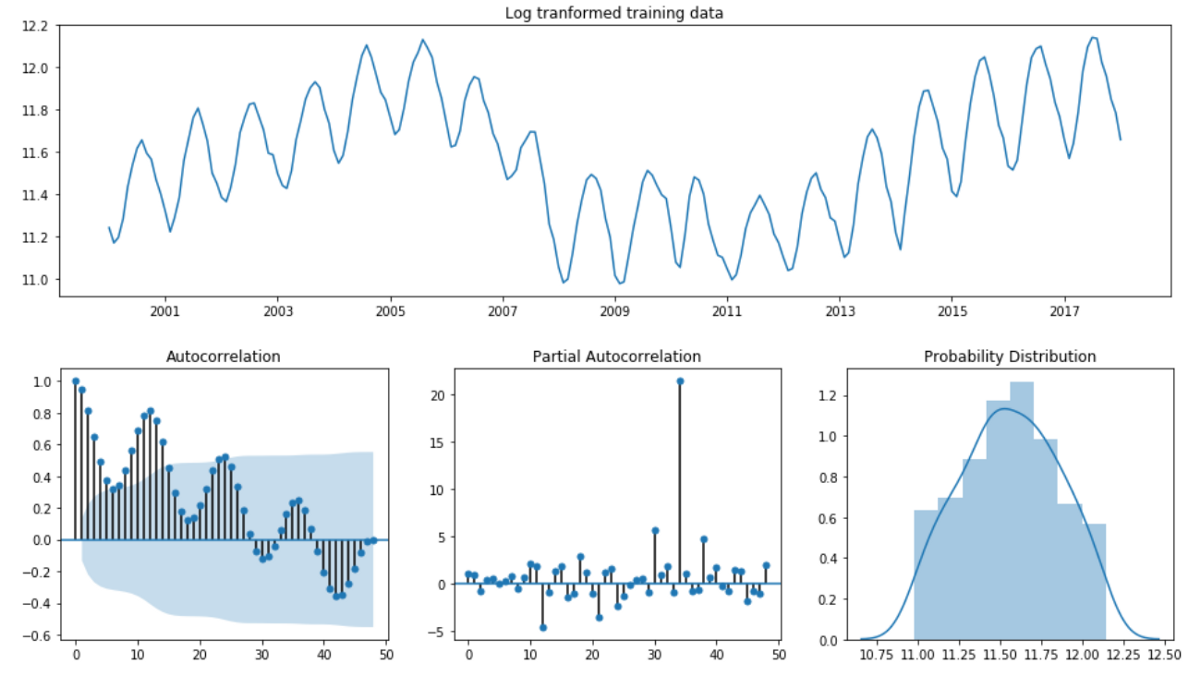## 原理

### 平稳过程

• 强平稳过程（Strict(ly) stationary process）：时间序列中给定长度的任意两段子序列联合概率分布相同。可以推知强平稳过程 $x_t$ 的期望和方差不随t变化；
• 弱平稳过程：时间序列满足均值平稳性（stationary in mean）和二阶平稳性（secondary order stationary）
• 均值平稳性：均值不变，与t无关
• 二阶平稳性：$X(t)$ 与 $X(t-k)$ 的协方差只与滞后阶数k有关，与t无关，即相关系数只取决于时间间隔而与时间起点无关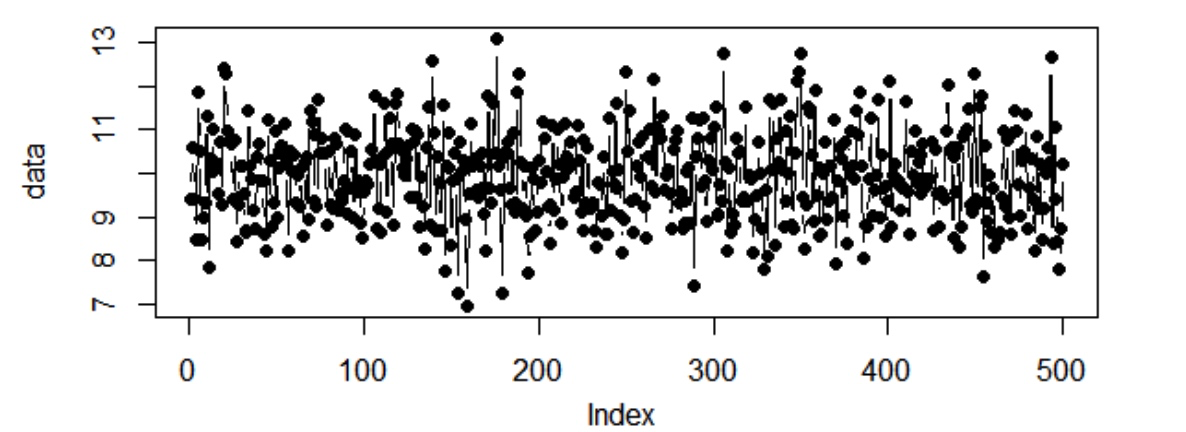### 自相关性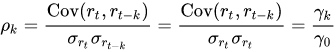ACF 自相关图：X(t) 时间序列与 X(t-1), X(t-2),.., X(t-k)各阶之后时间序列之间的相关系数与滞后阶数 k 的关系。

• 如果 k 阶滞后序列 X(t-k)与原始序列 X(t)的相关系数在 [-0.2, 0.2] 之间，则称 X(t-k)与 X(t)不具有相关性。如果一个时间序列的任何一阶滞后序列与原始序列都不具有相关性，则该时间序列不具有自相关性
• 白噪声序列：均值为 0，且不具有自相关性的平稳序列

### AR

p 阶自回归模型 $AR(p)$可以表示如下：

• $c$：常数项；
• $y_{t-k}$：前k期历史值；
• ${\varepsilon _{t}}$：白噪声；

• 当 $ϕ_1=0$ 时, $y_t$ 相当于白噪声；
• 当 $ϕ_1=1$ 并且 $c=0$ 时, $y_t$ 相当于随机游走模型；
• 当 $ϕ_1=1$ 并且 $c≠0$ 时, $y_t$ 相当于带漂移的随机游走模型；
• 当 $ϕ_1<0$ 时, $y_t$ 倾向于在正负值之间上下浮动;• 对于 $AR(1)$ 模型：$−1<ϕ1<1$；
• 对于 $AR(2)$ 模型：$−1<ϕ2<1$，$ϕ_1+ϕ_2<1$，$ϕ_2−ϕ_1<1$ 。
• 当 $p≥3$ 时，约束条件会更为复杂一些。R在估计自回归模型时可以解决这个问题

• 必须具有自相关，自相关系数（$\varphi _{i}$）是关键。如果自相关系数(R)小于0.5，则不宜采用，否则预测结果极不准确；
• 自回归只能适用于预测与自身前期相关的经济现象，即受自身历史因素影响较大的经济现象，如矿的开采量，各种自然资源产量等；对于受社会因素影响较大的经济现象，不宜采用自回归，而应改采可纳入其他变数的向量自回归模型；

### MA

• $c$：常量；
• $\varepsilon_t$ ：白噪声;• 对于MA(1)模型: $−1<θ_1<1$
• 对于MA(2)模型: $−1<θ_2<1$，$θ_2+θ_1>−1$，$θ_1−θ_2<1$
• 对于 $q≥3$的其他更复杂的情况，R会在在估计模型时解决这个问题

### 差分

$y_t$ 的 d 阶差分可以表示为：

## 实践

ARIMA 模型使用的一般流程：

1. 根据时间序列的散点图、自相关函数和偏自相关函数图识别其平稳性。
2. 对非平稳的时间序列数据进行平稳化处理。直到处理后的自相关函数和偏自相关函数的数值非显著非零。
3. 根据所识别出来的特征建立相应的时间序列模型。平稳化处理后，若偏自相关函数是截尾的，而自相关函数是拖尾的，则建立AR模型；若偏自相关函数是拖尾的，而自相关函数是截尾的，则建立MA模型；若偏自相关函数和自相关函数均是拖尾的，则序列适合ARMA模型。
4. 参数估计，检验是否具有统计意义。
5. 假设检验，判断（诊断）残差序列是否为白噪声序列。
6. 利用已通过检验的模型进行预测。

### 时间序列预处理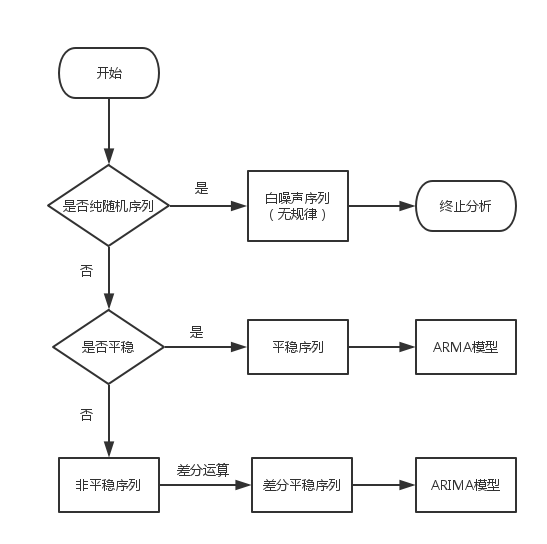#### 平稳性检验

1. 时序图检验：根据平稳时间序列的均值和方差都为常数的性质，平稳序列的时序图显示该序列值始终在一个常数附近随机波动，而且波动的范围有界；如果有明显的趋势性或者周期性，那它通常不是平稳序列
2. 自相关图检验：平稳序列具有短期相关性，这个性质表明对平稳序列而言通常只有近期的序列值对现时值得影响比较明显，间隔越远的过去值对现时值得影响越小。随着延迟期数k的增加，平稳序列的自相关系数会比较快的衰减趋向于零，并在零附近随机波动，而非平稳序列的自相关系数衰减的速度比较慢。
3. 单位根检验：最常用，原理待补充
4. 白噪声检验：一般是构造检验统计量来检验序列的纯随机性，常用的检验统计量有Q统计量、LB统计量，由样本各延迟期数的自相关系数可以计算得到检验统计量，然后计算出对应的p值，如果p值显著大于显著性水平a，则表示该序列不能拒绝纯随机的原假设，可以停止对该序列的分析

1. 取对数
2. 取差分

### 参数选择

#### 自相关图和偏自相关图• 截尾：自相关函数（ACF）或偏自相关函数（PACF）在某阶后在0附近随机波动
• 拖尾：始终有非零取值，不会在k大于某个常数后就恒等于零

1. AR模型：自相关系数拖尾，偏自相关系数截尾；
2. MA模型：自相关系数截尾，偏自相关函数拖尾；
3. ARMA模型：自相关函数和偏自相关函数均拖尾。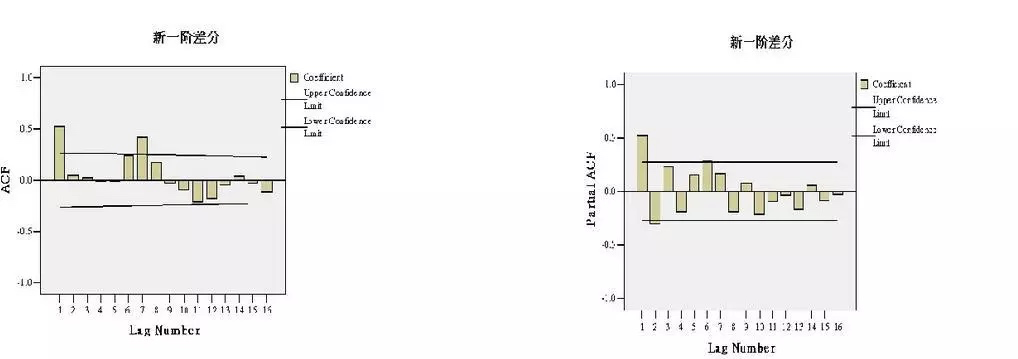#### 极大似然估计

AIC信息准则（Akaike Information Criterion）在选择用于回归模型的变量时非常有用， 同样在确定ARIMA模型阶数时也可以发挥很大作用。它可以写作:

BIC信息准则（Bayesian Information Criterion）的表示方式如下: